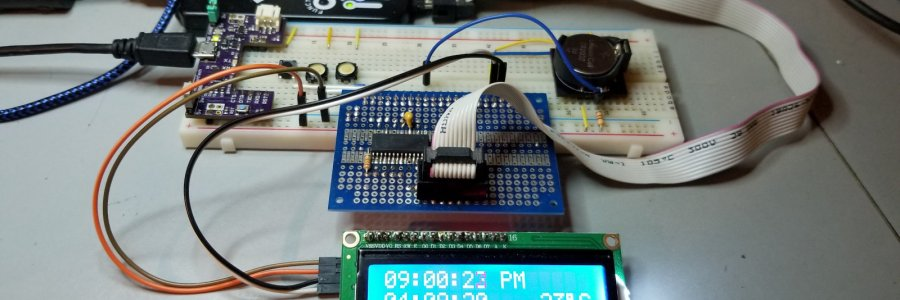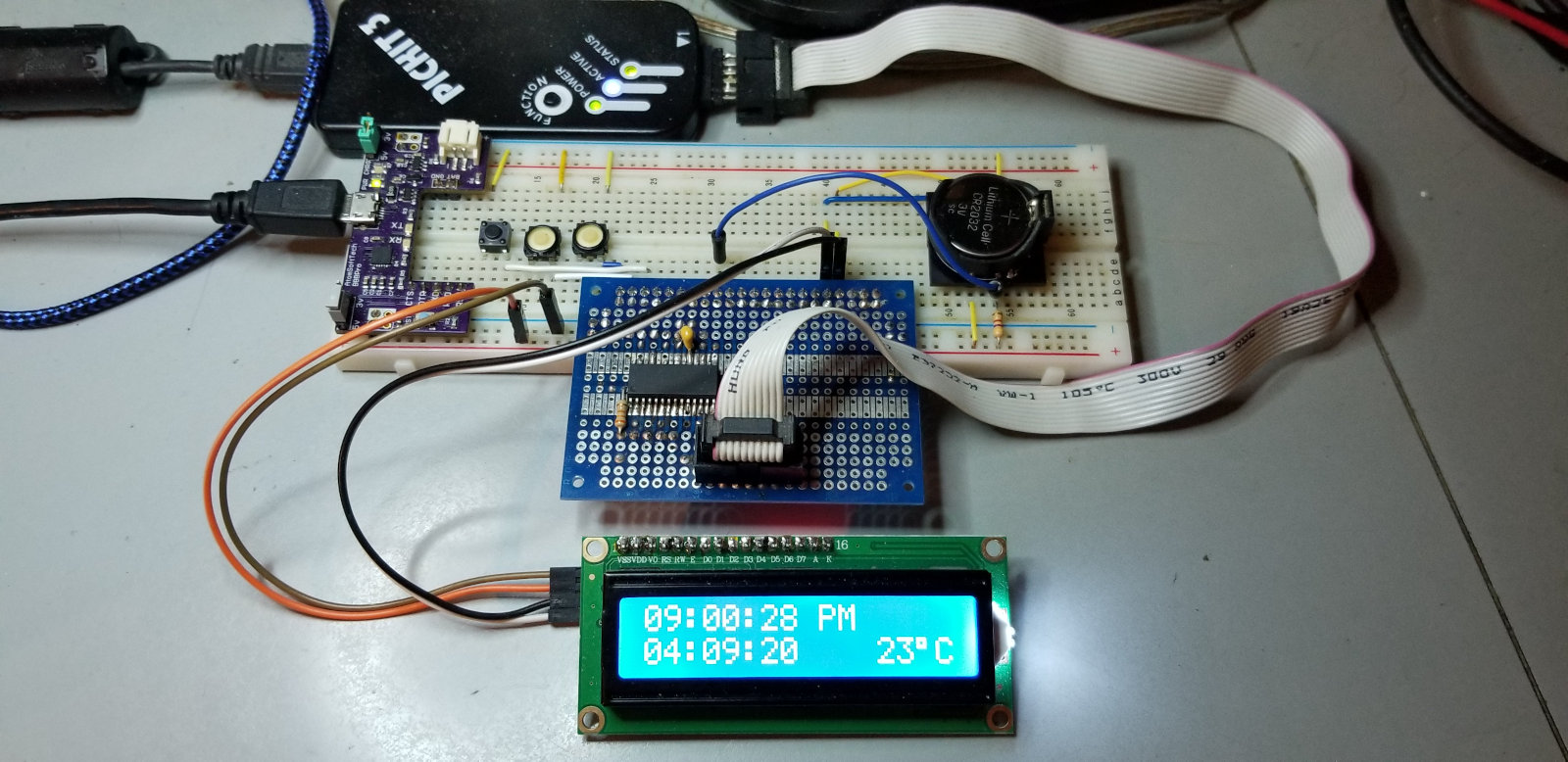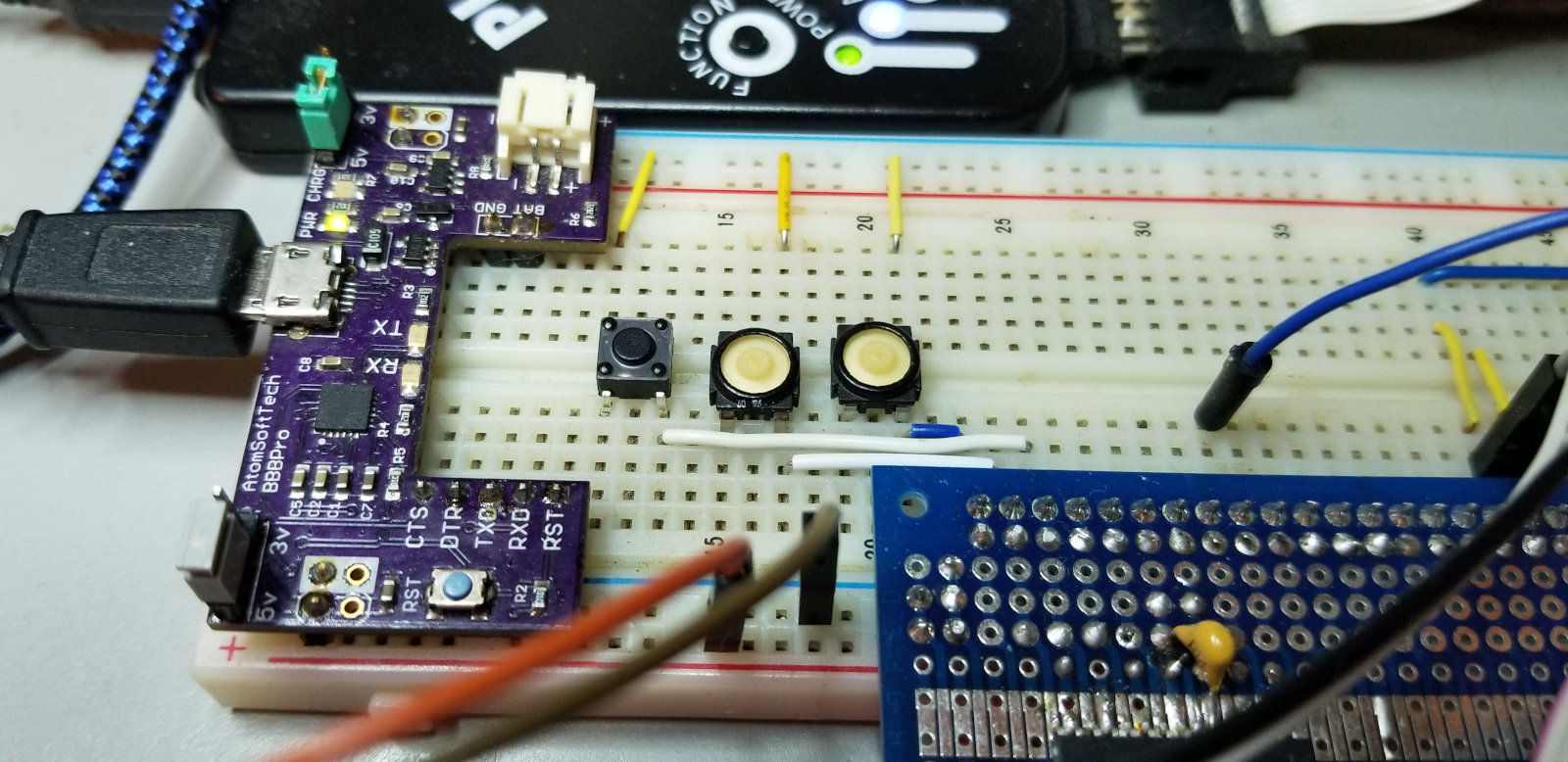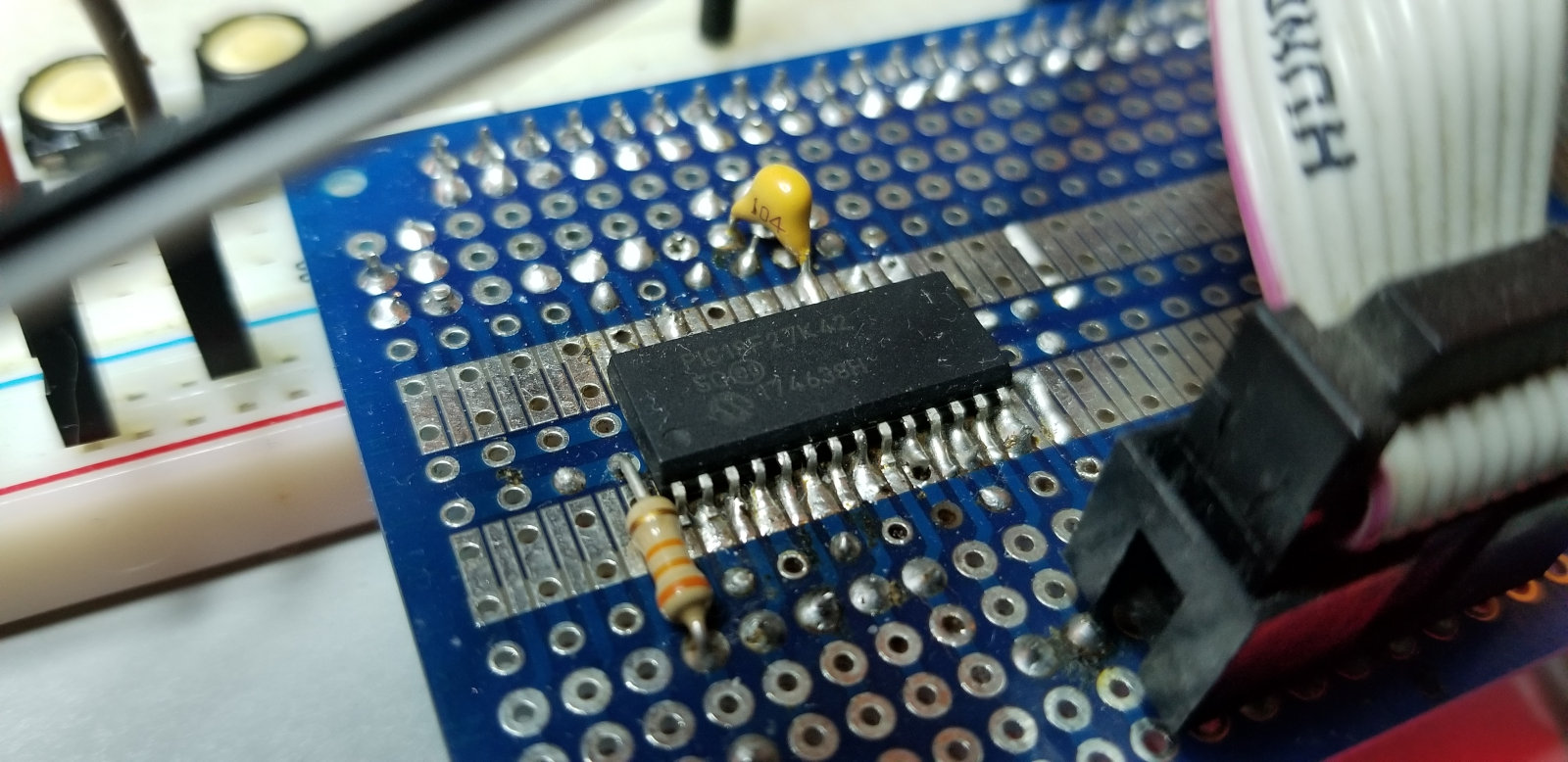#### 28-Pin PIC board - Part 4A whole lot of rewriting and changes has happened since the last post. It's far from complete, but what's there is fairly useable, I think. I'm a whole lot happier with this code than the last iteration. But that could change quickly. We shall see...

I cut i2c timings down from 20uS to 10uS, and that's far enough. Still far enough above minimums that I should have no problems with it. It works just fine so far.I cut the Timer0 interrupt to 61Hz and adjusted counters to suit. Works just fine still, and now spends half as much time in the ISR as before. Maybe I'll try it at even lower frequencies and see how it behaves. For now it works pretty well.

Removed all sprintf calls and wrote my own simple code to do the same things. That saved a ton of flash memory, and wasn't difficult to do. Sprintf is convenient, but so fat. Fine for PCs, but too porky for microcontrollers, unless your program is small and you have memory to burn.

I added a third button to make setting time and alarms easier.I'm now reading temperature from the DS3231 and displaying it. After tinkering with the fractional part of the temperature for a while I decided it wasn't necessary and was eating up display space, so it got dumped. Whole numbers only. Close enough.

I've started on alarm/watering time code. Alarm setting code has been added, though it is still far from complete, and the alarms do nothing still.I think I'll tinker with CVD (Capacitive Voltage Divider) capacitive touchpads instead of the buttons for controls. That functionality is built into the ADC module. I believe all IO pins can be used for ADC and so there's capability for lots of "buttons". I have no idea how to do it yet, but I'll figure it out.

I'll probably also add one or more rotary encoders for entering settings. Much nicer for spinning through lots of numbers quickly than buttons. Makes going back a number or six much easier and quicker. My current code has no way to back up. You have to keep on pushing that button until the number wraps around and do it again.

I'm waiting on a 20x4 LCD screen that I'll change to and rewrite for when it gets here. The 16x2 is a bit cramped, and requires multiple screens for setting things. Keeping everything on one screen would be nicer.

I also have a relay board coming, so I'll get that connected and controlling my irrigation pump. Then there will be a whole bunch of trial and error to find out how much on-time is necessary to do an every day, or every two days, hempy bucket nutrient water top-up without overflowing the hempy reservoirs and wasting expensive nutrient solution. I don't want to do sensing and make it smart - I mean, I'd love to, but I hear that nutrient salts corrode sensors to garbage very quickly. Dumb timer mode is going to work fine, I hope.

Later I might even switch to a nice color TFT screen. Just the 20x4 LCD for now though.

So much to do still...

#### lcd-i2c-rtc.c

(Scroll down for .h file)

``````#include "lcd-i2c-rtc.h"

#define dat   TRISCbits.TRISC4
#define clk   TRISCbits.TRISC3

unsigned char sec,sec10,min,min10,hour,hour10,ampm,date1,date10,mon,mon10,year1,year10;
unsigned char seconds,minutes,hours,date,month,year,junk;
unsigned char a1_minutes,a1_hours,a1_date;
unsigned char a2_minutes,a2_hours,a2_date;
unsigned char a1_min,a1_min10,a1_hour,a1_hour10,a1_ampm,a1_date1,a1_date10,a1_mon,a1_mon10,a1_year1,a1_year10;
unsigned char a2_min,a2_min10,a2_hour,a2_hour10,a2_ampm,a2_date1,a2_date10,a2_mon,a2_mon10,a2_year1,a2_year10;
signed char tempmsb,templsb;
char string;

unsigned char backlight = 0x08;   //backlight flag (bit3) enabled
int backlight_count = 0;

unsigned char button0_count = 0;  //button0 debounce counter
__bit button0_good = 0;           //button0 verified press flag

unsigned char button1_count = 0;  //button1 debounce counter
__bit button1_good = 0;           //button1 verified press flag

unsigned char button2_count = 0;  //button2 debounce counter
__bit button2_good = 0;           //button2 verified press flag

__bit refresh = 0;                //display refresh flag

__bit a1_onoff = 0;               //alarm1 on/off toggle
__bit a2_onoff = 0;

void __interrupt() bleh()
{
if(PIR3bits.TMR0IF && PIE3bits.TMR0IE){ //button0 pressed
if(PORTAbits.RA0 == 0){       //if button0 pressed
button0_count++;            //then increment button0 debounce counter
if(button0_count > 7)      //if 15 good button0 lows
button0_good = 1;         //then set button0 good press flag
}

if(PORTAbits.RA1 == 0){       //if button1 pressed
button1_count++;            //then increment button1 debounce counter
if(button1_count > 7)      //if 15 good button1 lows
button1_good = 1;         //then set button1 good press flag
}

if(PORTAbits.RA2 == 0){
button2_count++;
if(button2_count > 7)
button2_good = 1;
}

PIR3bits.TMR0IF = 0;          //clear timer0 interrupt flag
}

if(IOCBFbits.IOCBF0 && PIE0bits.IOCIE){ //1Hz rising edge from RTC
refresh = 1;                          //set display refresh flag
IOCBFbits.IOCBF0 = 0;                 //clear interrupt on change flag
}

if(backlight_count < 1500)      //around 23 seconds
backlight_count++;            //before backlight off
else{
backlight_count = 0;
backlight = 0x00;
}
}

void main()
{
int count;

INTCON0bits.IPEN = 0;   //priority ints disabled
T0CON1 = 0x42;          //timer0 fosc/4, prescale 1:4
T0CON0 = 0x90;          //timer0 on, 16 bit, no postscale
PIE3bits.TMR0IE = 1;    //TMR0 interrupt enabled

IOCBPbits.IOCBP0 = 1;   //interrupt on change for RB0 enabled
PIE0bits.IOCIE = 1;     //interrupt on change enabled

TRISA = 0x07;           //porta all outs except bit 0, 1, 2
ANSELA = 0;             //porta all digital
WPUA = 0x07;            //porta weak pullups enabled on 0, 1, 2

TRISB = 0x01;           //portb R0 is input
ANSELB = 0;             //portb all digital

TRISC = 0b00011000;     //portc all outs except i2c
LATC = 0;               //portc all zeros
ANSELC = 0;             //portc all digital
__delay_ms(5);

lcd_init();
alarmclean();           //overwrite garbage in alarm regs
INTCON0bits.GIE = 1;    //global ints enabled

while(1){
if(refresh){
display_refresh();
refresh = 0;
}

if(button0_good){
setclock();
button0_good = 0;
button0_count = 0;
}

if(button2_good){
setalarm();
button2_good = 0;
button0_count = 0;
}

if(button1_good){
backlight_count = 0;
backlight = 0x08;
button1_good = 0;
button1_count = 0;
}
}
}

void display_refresh(){
unsigned char bleh,dig1,dig2;
i2c_write(0xd0);
i2c_write(0x00);
restart();
i2c_write(0xd1);

junk = i2c_read();            //alarm 1 sec (unused)
junk = i2c_read();            //alarm 1 day (unused)

junk = i2c_read();            //alarm 2 day (unused)

i2c_write(0xd0);
i2c_write(0x11);
restart();
i2c_write(0xd1);
stop();

sec = (seconds & 0x0f) + 0x30;           //prep data for display
sec10 = ((seconds & 0x70) >> 4) + 0x30;
min = (minutes & 0x0f) + 0x30;
min10 = ((minutes & 0x70) >> 4) + 0x30;
hour = (hours & 0x0f) + 0x30;
hour10 = ((hours & 0x10) >> 4) + 0x30;
ampm = (hours & 0x20) >> 5;
date1 = (date & 0x0f) + 0x30;
date10 = ((date & 0x30) >> 4) + 0x30;
mon = (month & 0x0f) + 0x30;
mon10 = ((month & 0x10) >> 4) + 0x30;
year1 = (year & 0x0f) + 0x30;
year10 = ((year & 0xf0) >> 4) + 0x30;
dig1 = (tempmsb / 10) + 0x30;
dig2 = (tempmsb % 10) + 0x30;

start();                        //display data
i2c_write(0x4e);                //send lcd slave address & write bit
lcd_cmd(0x80);                  //line 1
lcd_char(hour10);
lcd_char(hour);
lcd_char(0x3a);                 //colon
lcd_char(min10);
lcd_char(min);
lcd_char(0x3a);
lcd_char(sec10);
lcd_char(sec);
lcd_char(0x20);                 //space
if(ampm)
lcd_string("PM");
else
lcd_string("AM");

lcd_cmd(0xc0);                  //line 2
lcd_char(mon10);
lcd_char(mon);
lcd_char(0x3a);                 //colon
lcd_char(date10);
lcd_char(date1);
lcd_char(0x3a);
lcd_char(year10);
lcd_char(year1);
lcd_string("    ");             //spaces
lcd_char(dig1);                 //temperature
lcd_char(dig2);
lcd_char(0xdf);                 //degrees
lcd_char(0x43);                 //C
stop();
}

void setalarm(){
unsigned char digit = 1;
static __bit done;
done = 1;
backlight = 0x08;                 //backlight on

a1_min = (a1_minutes & 0x0f) + 0x30;
a1_min10 = ((a1_minutes & 0x70) >> 4) + 0x30;
a1_hour = (a1_hours & 0x0f) + 0x30;
a1_hour10 = ((a1_hours & 0x10) >> 4) + 0x30;
a1_ampm = (a1_hours & 0x20) >> 5;
a1_date1 = (a1_date & 0x0f) + 0x30;
a1_date10 = ((a1_date & 0x30) >> 4) + 0x30;

a2_min = (a2_minutes & 0x0f) + 0x30;
a2_min10 = ((a2_minutes & 0x70) >> 4) + 0x30;
a2_hour = (a2_hours & 0x0f) + 0x30;
a2_hour10 = ((a2_hours & 0x10) >> 4) + 0x30;
a2_ampm = (a2_hours & 0x20) >> 5;
a2_date1 = (a2_date & 0x0f) + 0x30;
a2_date10 = ((a2_date & 0x30) >> 4) + 0x30;

lcd_cls();

while(done){                      //display alarm1 set data
start();
i2c_write(0x4e);                //send lcd slave address & write bit
lcd_cmd(0x80);                  //line 1

lcd_string("A1  ");
else{
lcd_string("A1 ");
lcd_char(a1_hour10);
}

lcd_string(" :");
else{
lcd_char(a1_hour);
lcd_char(0x3a);
}

lcd_char(0x20);
else
lcd_char(a1_min10);

lcd_string(" :");
else{
lcd_char(a1_min);
lcd_char(0x3a);
}

lcd_string("  ");
else{
if(a1_ampm)
lcd_string("PM");
else
lcd_string("AM");
}

lcd_string("     ");
else{
if(a1_onoff)
lcd_string("   ON");
else
lcd_string("  OFF");
}

lcd_cmd(0xc0);                  //line 2

lcd_string("Day of month  ");
else{
lcd_string("Day of month ");
lcd_char(a1_date10);
}

lcd_char(0x20);
else
lcd_char(a1_date1);

stop();

if(button0_good){
++digit;
button0_count = 0;
button0_good = 0;
}

if(button1_good){
switch(digit){
case 1:
a1_hour++;
if(a1_hour > 0x39){
a1_hour = 0x30;
a1_hour10 = 0x31;
}
if(a1_hour10 == 0x31 && a1_hour > 0x32){
a1_hour = 0x31;
a1_hour10 = 0x30;
}
break;
case 2:
a1_min++;
if(a1_min > 0x39){
a1_min = 0x30;
a1_min10++;
if(a1_min10 > 0x35)
a1_min10 = 0x30;
}
if(a1_min10 > 0x35 && a1_min > 0x39){
a1_min = 0x30;
a1_min10 = 0x30;
}
break;
case 3:
a1_ampm = !a1_ampm;
break;
case 4:
a1_onoff = !a1_onoff;
case 5:
a1_date1++;
if(a1_date10 == 0x33 && a1_date1 > 0x31){
a1_date1 = 0x31;
a1_date10 = 0x30;
}
else if(a1_date1 > 0x39){
a1_date1 = 0x31;
a1_date10++;
}
break;
}
button1_good = 0;
button1_count = 0;
}

if(digit == 6){
__delay_ms(50);
done = 0;
}

else

backlight_count = 0;
__delay_ms(200);
}

a1_minutes = a1_min10 - 0x30;
a1_minutes <<= 4;
a1_minutes = a1_minutes | a1_min - 0x30;

a1_hours = a1_hour10 - 0x30;        //high digit
a1_hours <<= 4;                     //shift it into place
digit = ampm;                       //move am/pm bit into place
digit <<= 5;
a1_hours = a1_hours | 0x40;         //12 hour time bit
a1_hours = a1_hours | digit;        //am/pm bit
a1_hours = a1_hours | hour - 0x30;  //low digit

a1_date = a1_date10 - 0x30;
a1_date <<= 4;
a1_date = a1_date | a1_date1 - 0x30;

start();                //set alarm1
i2c_write(0xd0);        //send ID code & write bit
i2c_write(a1_minutes);  //\$00 set alarm1 minutes
i2c_write(a1_hours);    //\$01 set alarm1 hours
i2c_write(a1_date);     //\$02 set alarm1 date
i2c_write(a2_minutes);  //\$03 set alarm2 minutes
i2c_write(a2_hours);    //\$04 set alarm2 hours
i2c_write(a2_date);     //\$06 set alarm2 date
stop();

lcd_cls();
}

void setclock(){
unsigned char digit = 0;
static __bit done;
done = 1;
backlight = 0x08;                 //backlight on

//Clock Set
while(done){                      //display clock set data
start();
i2c_write(0x4e);                //send lcd slave address & write bit
lcd_cmd(0x80);                  //line 1

lcd_char(0x20);
else{
lcd_char(hour10);
}

lcd_string(" :");
else{
lcd_char(hour);
lcd_char(0x3a);
}

lcd_char(0x20);
else{
lcd_char(min10);
}

lcd_string(" :");
else{
lcd_char(min);
lcd_char(0x3a);
}

lcd_char(0x20);
else{
lcd_char(sec10);
}

lcd_string("  ");
else{
lcd_char(sec);
lcd_char(0x20);
}

lcd_string("  ");
else{
if(ampm)
lcd_string("PM");
else
lcd_string("AM");
}

lcd_cmd(0xc0);                  //line 2

lcd_char(0x20);
else{
lcd_char(mon10);
}

lcd_string(" :");
else{
lcd_char(mon);
lcd_char(0x3a);
}

lcd_char(0x20);
else{
lcd_char(date10);
}

lcd_string(" :");
else{
lcd_char(date1);
lcd_char(0x3a);
}

lcd_string("20 ");
else{
lcd_string("20");
lcd_char(year10);
}

lcd_char(0x20);
else{
lcd_char(year1);
}
stop();

if(button0_good){
++digit;
button0_count = 0;
button0_good = 0;
}

if(button1_good){
switch(digit){
case 1:
hour++;
if(hour > 0x39){
hour = 0x30;
hour10 = 0x31;
}
if(hour10 == 0x31 && hour > 0x32){
hour = 0x31;
hour10 = 0x30;
}
break;
case 2:
min++;
if(min > 0x39){
min = 0x30;
min10++;
if(min10 > 0x35)
min10 = 0x30;
}
if(min10 > 0x35 && min > 0x39){
min = 0x30;
min10 = 0x30;
}
break;
case 3:
sec = 0x30;
sec10 = 0x30;
break;
case 4:
ampm = !ampm;
break;
case 5:
mon++;
if(mon > 0x39){
mon = 0x30;
mon10 = 0x31;
}
if(mon10 == 0x31 && mon > 0x32){
mon = 0x31;
mon10 = 0x30;
}
break;
case 6:
date1++;
if(date10 == 0x33 && date1 > 0x31){
date1 = 0x31;
date10 = 0x30;
}
else if(date1 > 0x39){
date1 = 0x31;
date10++;
}
break;
case 7:
year1++;
if(year1 > 0x39){
year1 = 0x30;
year10++;
if(year10 > 0x39)
year10 = 0x30;
}
if(year10 == 0x39 && year1 > 0x39){
year1 = 0x30;
year10 = 0x30;
}
}
button1_good = 0;
button1_count = 0;
}

if(digit == 8){
__delay_ms(50);
done = 0;
}

else

backlight_count = 0;
__delay_ms(200);
}

seconds = sec10 - 0x30;          //prep new data for clock setting
seconds <<= 4;
seconds = seconds | sec - 0x30;

minutes = min10 - 0x30;
minutes <<= 4;
minutes = minutes | min - 0x30;

hours = hour10 - 0x30;           //high digit
hours <<= 4;              //shift it into place
digit = ampm;             //move am/pm bit into place
digit <<= 5;
hours = hours | 0x40;     //12 hour time bit
hours = hours | digit;    //am/pm bit
hours = hours | hour - 0x30;     //low digit

month = mon10 - 0x30;
month <<= 4;
month = month | mon - 0x30;

date = date10 - 0x30;
date <<= 4;
date = date | date1 - 0x30;

year = year10 - 0x30;
year <<= 4;
year = year | year1 - 0x30;

start();                //set the clock
i2c_write(0xd0);        //send ID code & write bit
i2c_write(seconds);     //\$00 set seconds
i2c_write(minutes);     //\$01 set minutes
i2c_write(hours);       //\$02 set hours
i2c_write(junk);        //\$03 set day
i2c_write(date);        //\$04 set date
i2c_write(month);       //\$05 set month
i2c_write(year);        //\$06 set year
restart();
i2c_write(0xd0);        //ID code & write bit
i2c_write(0x40);        //enable 1Hz square wave
stop();

lcd_cls();
}

//* I2C functions *
//*****************
unsigned char i,tmp = 0;
static __bit mbit = 0;
__delay_us(10);
for(i=0;i<8;i++){
dat = 1;            //sda high
__delay_us(10);      //minimum clock low time
clk = 1;            //clk high
__delay_us(5);       //1/2 min clock high time
mbit = PORTCbits.RC4;     //read the data bit
if(mbit)            //store it in tmp
tmp = tmp | 0x01;
__delay_us(5);       //last 1/2 min clock high time
if(i < 7)
tmp <<= 1;        //shift left for next bit
clk = 0;            //lower clock
__delay_us(10);      //minimum clock low time
}
PORTC = 0;
dat = 0;              //send ACK
__delay_us(10);        //data settle time
i2c_clock();          //pulse the clock
dat = 1;              //release ACK
__delay_us(10);        //gap between next byte
return(tmp);
}

unsigned char i,tmp = 0;
static __bit mbit;
__delay_us(10);
for(i=0;i<8;i++){
dat = 1;              //SDA = input
__delay_us(10);       //minimum clock low time
clk = 1;              //release clock
__delay_us(5);        //1/2 min clock high time
mbit = PORTCbits.RC4;     //read the data bit
if(mbit)              //store it in tmp
tmp = tmp | 0x01;
__delay_us(5);        //last 1/2 min clock high time
if(i < 7)
tmp <<= 1;          //shift left for next bit
clk = 0;              //lower clock
__delay_us(10);       //minimum clock low time
}
PORTCbits.RC4 = 0;
dat = 1;                //send NACK
__delay_us(10);         //data settle time
i2c_clock();            //pulse the clock
return(tmp);
}

unsigned char i2c_write(unsigned char x){
unsigned char i;
__delay_us(10);
for(i=0;i<8;i++){
dat = 0;          //set data bit low
if(x &  0x80)     //if output bit is high
dat = 1;        //then set data bit high
i2c_clock();      //clock it out
x <<= 1;          //shift next bit into position
}
//get ack
dat = 1;            //set data high (input)
PORTCbits.RC4 = 0;  //clear data bit
clk = 1;            //set clock high
__delay_us(5);      //wait half a clock pulse
if(PORTCbits.RC4)   //sample data bit
return(1);        //nack error
__delay_us(5);      //ack good, wait other half of clock pulse
clk = 0;            //set clock low
__delay_us(10);
dat = 1;            //set data
return(0);
}

void start(void){     //send start condition
dat = 0;            //set data low
__delay_us(10);
clk = 0;
__delay_us(10);
}

void restart(void){     //send start condition
dat = 1;              //release data
__delay_us(10);
clk = 1;
__delay_us(10);
dat = 0;
__delay_us(10);
clk = 0;
__delay_us(10);
}

void stop(void){      //send stop condition
dat = 0;            //set data low
__delay_us(10);
clk = 1;            //set clock high
__delay_us(10);       //stop delay
dat = 1;            //set data high
__delay_us(10);
}

void i2c_clock(void){
clk = 1;            //set clock high
__delay_us(10);
clk = 0;            //set clock low
__delay_us(10);
}

//* LCD functions *
//*****************
void lcd_cls(void){
start();
i2c_write(0x4e);                //send lcd slave address & write bit
lcd_cmd(0x80);                  //line 1
lcd_string("                ");
lcd_cmd(0xc0);                  //line 2
lcd_string("                ");
stop();
__delay_us(5000);
}

void lcd_string(char *senpoint)
{
while(*senpoint != '\0')
{
lcd_char(*senpoint);
senpoint++;
}
}

void lcd_cmd(unsigned char letter)
{
unsigned char temp;
temp = letter;
temp = temp & 0xf0;       //clear lower nybble
temp = temp | 0b00000100; //set E, RW = write, RS = command
temp = temp | backlight;  //set backlight on or off
i2c_write(temp);          //send upper nybble
temp = temp & 0b11110000; //clear E to latch data and resend
temp = temp | backlight;  //set backlight on or off
i2c_write(temp);

temp = letter;
temp = temp & 0x0f;       //clear upper nybble
temp = temp << 4;         //shift left 4 bits
temp = temp | 0b00000100; //send lower nybble
temp = temp | backlight;  //set backlight on or off
i2c_write(temp);
temp = temp & 0b11110000;
temp = temp | backlight;  //set backlight on or off
i2c_write(temp);
}

void lcd_char(unsigned char letter)
{
unsigned char temp;
temp = letter;
temp = temp & 0xf0;       //clear lower nybble
temp = temp | 0b00000101; //set E, RW = write, RS = data
temp = temp | backlight;  //set backlight on or off
i2c_write(temp);          //send upper nybble
temp = temp & 0b11110001; //clear E to latch data and resend
temp = temp | backlight;  //set backlight on or off
i2c_write(temp);

temp = letter;
temp = temp & 0x0f;       //clear upper nybble
temp = temp << 4;         //shift left 4 bits
temp = temp | 0b00000101; //send lower nybble
temp = temp | backlight;  //set backlight on or off
i2c_write(temp);
temp = temp & 0b11110001;
temp = temp | backlight;  //set backlight on or off
i2c_write(temp);
}

void lcd_nybble(unsigned char nyb)
{
nyb = nyb << 4;           //shift left 4 bits
nyb = nyb | 0b00001100;   //send lower nybble
i2c_write(nyb);
nyb = nyb & 0b11111000;
i2c_write(nyb);
}

void lcd_init(void)
{
start();
i2c_write(0x4e);          //send lcd slave address & write bit
__delay_ms(50);
lcd_nybble(0x03);
__delay_ms(5);
lcd_nybble(0x03);
__delay_us(160);
lcd_nybble(0x03);
__delay_us(160);
lcd_nybble(0x02);
__delay_us(160);
lcd_cmd(0x28);            //set 4-bit mode and 2 lines
__delay_us(160);
lcd_cmd(0x10);            //cursor move & shift left
__delay_us(160);
lcd_cmd(0x06);            //entry mode = increment
__delay_us(160);
lcd_cmd(0b00001100);      //display on - cursor & blink off
__delay_us(160);
lcd_cmd(0x01);            //clear display
__delay_us(160);
stop();
}

void alarmclean(){        //clear alarm settings
unsigned char x;
start();
i2c_write(0xd0);        //send ID code & write bit
i2c_write(0);           //alarm1 seconds
i2c_write(0);           //alarm1 minutes
i2c_write(0x40);        //alarm1 hours
i2c_write(0);           //alarm1 date

i2c_write(0);           //alarm2 minutes
i2c_write(0x40);        //alarm2 hours
i2c_write(0);           //alarm2 date
stop();
}``````

#### lcd-i2c-rtc.h

``````// CONFIG1L
#pragma config FEXTOSC = ECH    // External Oscillator Selection (EC (external clock) above 8 MHz; PFM set to high power)
#pragma config RSTOSC = HFINTOSC_64MHZ// Reset Oscillator Selection (HFINTOSC with HFFRQ = 64 MHz and CDIV = 1:1)

// CONFIG1H
#pragma config CLKOUTEN = OFF   // Clock out Enable bit (CLKOUT function is disabled)
#pragma config PR1WAY = ON      // PRLOCKED One-Way Set Enable bit (PRLOCK bit can be cleared and set only once)
#pragma config CSWEN = ON       // Clock Switch Enable bit (Writing to NOSC and NDIV is allowed)
#pragma config FCMEN = ON       // Fail-Safe Clock Monitor Enable bit (Fail-Safe Clock Monitor enabled)

// CONFIG2L
#pragma config MCLRE = EXTMCLR  // MCLR Enable bit (If LVP = 0, MCLR pin is MCLR; If LVP = 1, RE3 pin function is MCLR )
#pragma config PWRTS = PWRT_OFF // Power-up timer selection bits (PWRT is disabled)
#pragma config MVECEN = OFF     // Multi-vector enable bit (Multi-vector disabled, Vector table not used for interrupts)
#pragma config IVT1WAY = ON     // IVTLOCK bit One-way set enable bit (IVTLOCK bit can be cleared and set only once)
#pragma config LPBOREN = OFF    // Low Power BOR Enable bit (ULPBOR disabled)
#pragma config BOREN = SBORDIS  // Brown-out Reset Enable bits (Brown-out Reset enabled , SBOREN bit is ignored)

// CONFIG2H
#pragma config BORV = VBOR_2P45 // Brown-out Reset Voltage Selection bits (Brown-out Reset Voltage (VBOR) set to 2.45V)
#pragma config ZCD = OFF        // ZCD Disable bit (ZCD disabled. ZCD can be enabled by setting the ZCDSEN bit of ZCDCON)
#pragma config PPS1WAY = ON     // PPSLOCK bit One-Way Set Enable bit (PPSLOCK bit can be cleared and set only once; PPS registers remain locked after one clear/set cycle)
#pragma config STVREN = ON      // Stack Full/Underflow Reset Enable bit (Stack full/underflow will cause Reset)
#pragma config DEBUG = OFF      // Debugger Enable bit (Background debugger disabled)
#pragma config XINST = OFF      // Extended Instruction Set Enable bit (Extended Instruction Set and Indexed Addressing Mode disabled)

// CONFIG3L
#pragma config WDTCPS = WDTCPS_31// WDT Period selection bits (Divider ratio 1:65536; software control of WDTPS)
#pragma config WDTE = OFF       // WDT operating mode (WDT Disabled; SWDTEN is ignored)

// CONFIG3H
#pragma config WDTCWS = WDTCWS_7// WDT Window Select bits (window always open (100%); software control; keyed access not required)
#pragma config WDTCCS = SC      // WDT input clock selector (Software Control)

// CONFIG4L
#pragma config BBSIZE = BBSIZE_512// Boot Block Size selection bits (Boot Block size is 512 words)
#pragma config BBEN = OFF       // Boot Block enable bit (Boot block disabled)
#pragma config SAFEN = OFF      // Storage Area Flash enable bit (SAF disabled)
#pragma config WRTAPP = OFF     // Application Block write protection bit (Application Block not write protected)

// CONFIG4H
#pragma config WRTB = OFF       // Configuration Register Write Protection bit (Configuration registers (300000-30000Bh) not write-protected)
#pragma config WRTC = OFF       // Boot Block Write Protection bit (Boot Block (000000-0007FFh) not write-protected)
#pragma config WRTD = OFF       // Data EEPROM Write Protection bit (Data EEPROM not write-protected)
#pragma config WRTSAF = OFF     // SAF Write protection bit (SAF not Write Protected)
#pragma config LVP = OFF        // Low Voltage Programming Enable bit (HV on MCLR/VPP must be used for programming)

// CONFIG5L
#pragma config CP = OFF         // PFM and Data EEPROM Code Protection bit (PFM and Data EEPROM code protection disabled)

// CONFIG5H

//-------------------------------------------

#include <xc.h>
#include <stdio.h>

#define _XTAL_FREQ  64000000

void __interrupt() bleh(void);
void start(void);
void restart(void);
void stop(void);
void ack(void);
void nack(void);
void i2c_clock(void);
unsigned char i2c_write(unsigned char);